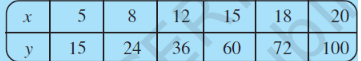# Q1. Observe the below table and find if x and y are directly proportional.Calculating   we get:

So, clearly  equals to   hence it is not equal to some constant value 'k'.

We can say that x and y are not directly proportional.

Exams
Articles
Questions